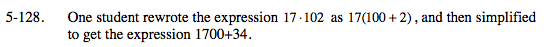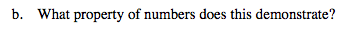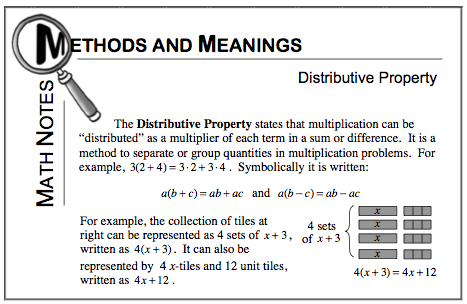### Home > MC2 > Chapter 5 > Lesson 5.3.4 > Problem5-128

5-128.
1. One student rewrote the expression 17 · 102 as 17(100 + 2), and then simplified to get the expression 1700 + 34. Homework Help ✎

2. What property of numbers does this demonstrate?Do you get the same answer when you evaluate all three equations?

17 (102) = 17 (100 + 2) = 1700 + 34

Is this true?Do you recall what property allows you to distribute a multiplier of each term in a sum?